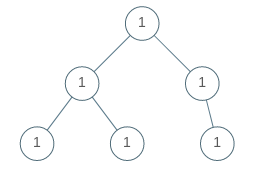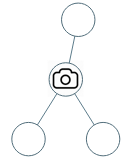# LeetCode Weekly Contest 117

https://leetcode.com/contest/weekly-contest-117

## Univalued Binary Tree

A binary tree is univalued if every node in the tree has the same value.

Return true if and only if the given tree is univalued.

Example 1:Example 2:Note:

1. The number of nodes in the given tree will be in the range [1, 100].
2. Each node’s value will be an integer in the range [0, 99].

BFS遍历所有值，找到与根结点不同的返回false

## Numbers With Same Consecutive Differences

Return all non-negative integers of length N such that the absolute difference between every two consecutive digits is K.

Note that every number in the answer must not have leading zeros except for the number 0 itself. For example, 01 has one leading zero and is invalid, but 0 is valid.

You may return the answer in any order.

Example 1:

Example 2:

Note:

1. 1 <= N <= 9
2. 0 <= K <= 9

N=1时，无论K取多少，连续位之间的绝对差为K始终成立，因此0-9所有个位数都成立。N每增加1，需要对N-1时成立的所有结果，添加满足连续位差值的最后一位，该位取值如果还在0-9之间则放入新的结果中，如果第一位为0则不需要考虑，直接舍弃。需要注意如果K=0时只需要考虑一种添加末位的情况，避免重复计数。

## Vowel Spellchecker

Given a wordlist, we want to implement a spellchecker that converts a query word into a correct word.

For a given query word, the spell checker handles two categories of spelling mistakes:

• Capitalization: If the query matches a word in the wordlist (case-insensitive), then the query word is returned with the same case as the case in the wordlist.
• Example: wordlist = ["yellow"], query = "YellOw": correct = "yellow"
• Example: wordlist = ["Yellow"], query = "yellow": correct = "Yellow"
• Example: wordlist = ["yellow"], query = "yellow": correct = "yellow"
• Vowel Errors: If after replacing the vowels (‘a’, ‘e’, ‘i’, ‘o’, ‘u’) of the query word with any vowel individually, it matches a word in the wordlist (case-insensitive), then the query word is returned with the same case as the match in the wordlist.
• Example: wordlist = ["YellOw"], query = "yollow": correct = "YellOw"
• Example: wordlist = ["YellOw"], query = "yeellow": correct = "" (no match)
• Example: wordlist = ["YellOw"], query = "yllw": correct = "" (no match)

In addition, the spell checker operates under the following precedence rules:

• When the query exactly matches a word in the wordlist (case-sensitive), you should return the same word back.
• When the query matches a word up to capitlization, you should return the first such match in the wordlist.
• When the query matches a word up to vowel errors, you should return the first such match in the wordlist.
• If the query has no matches in the wordlist, you should return the empty string.

Given some queries, return a list of words answer, where answer[i] is the correct word for query = queries[i].

Example 1:

Note:

1. 1 <= wordlist.length <= 5000
2. 1 <= queries.length <= 5000
3. 1 <= wordlist[i].length <= 7
4. 1 <= queries[i].length <= 7
5. All strings in wordlist and queries consist only of english letters.

## Binary Tree Cameras

Given a binary tree, we install cameras on the nodes of the tree.

Each camera at a node can monitor its parent, itself, and its immediate children.

Calculate the minimum number of cameras needed to monitor all nodes of the tree.

Example 1:Example 2:Note:

1. The number of nodes in the given tree will be in the range [1, 1000].
2. Every node has value 0.

• 如果一个结点的两个子结点有一个还没被监控覆盖，那么这个结点就需要装上摄像头，成为2
• 如果两个子结点有一个装有摄像头，另一个也不需要再装摄像头来覆盖（12），那么该结点已经被监控覆盖，标记为1
• 如果两个子结点都已经被它们的子结点的摄像头覆盖监控了，那么当前结点不需要装监控来向下覆盖，但也没被覆盖，标记为0